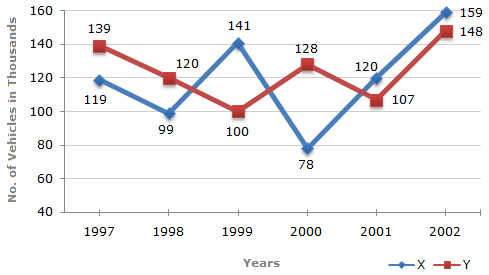# Data Interpretation - Line Charts - Discussion

### Discussion :: Line Charts - Line Chart 3 (Q.No.3)

Study the following line graph and answer the questions based on it.

Number of Vehicles Manufactured by Two companies ove the Years (Number in Thousands)3.

What is the average numbers of vehicles manufactured by Company X over the given period ? (rounded off to nearest integer)

 [A]. 119333 [B]. 113666 [C]. 112778 [D]. 111223

Explanation:

Average number of vehicles manufactured by Company X

 = 1 x (119000 + 99000 + 141000 + 78000 + 120000 + 159000) 6

= 119333.

 Ano said: (Jun 21, 2016) There are 5 years! how can you divide it by 6.

 Abhilasha Mishra said: (Sep 14, 2016) There are 6 numbers. So, we have to divide 6. Am I right?

 Saikumar said: (Jan 4, 2017) There are six years, and 6 different values. Don't consider 97-98, 98-99 and so on as number of years, for each and every year there is definite value.